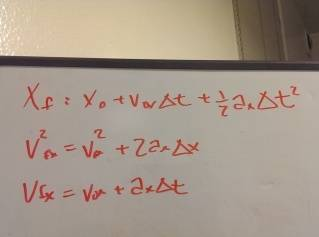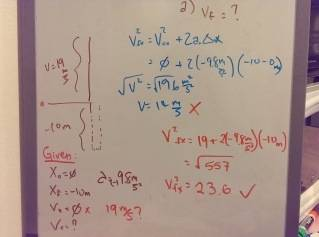# Finding final velocity

I am not good at typing out formulas and equations on the internet, so I took pictures of what I did instead. I hope that's not too informal.

1. Homework Statement

A rock is tossed straight up with a velocity of 19 m/s. When it returns it fell into a 10 m deep hole.

Q: What is the rock's velocity as it hits the bottom of the hole?

## Homework Equations## The Attempt at a SolutionSo in the end I figure out that I am suppose to plug 19 m/s as my initial velocity, but I don't get why.
If the rock is tossed straight up should it not means that the initial velocity = 0 because initially the rock is at rest?[/B]

SteamKing
Staff Emeritus
Homework Helper
According to the OP, "A rock is tossed straight up with a velocity of 19 m/s."

You can't write a plainer statement than that. IDK how you could assume that the rock is at rest, which implies no movement of any kind.

phinds